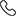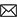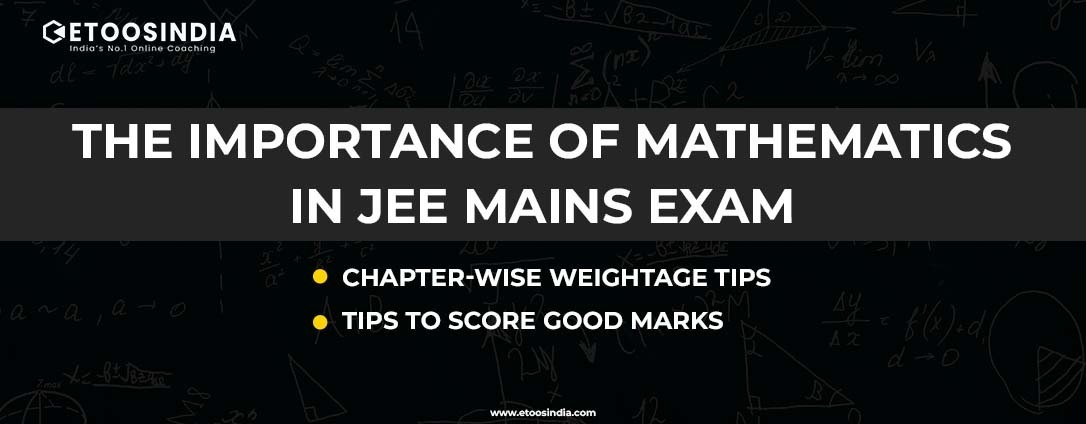Sales :9214233303 / Tech. Support Desk :9214233360/40 (9AM ~ 6PM)# Importance Of Mathematics In JEE Mains Exam | Tips To Score Good Marks

06 Oct, 2022, By Tanvi SharmaAre you focusing on getting admission to the top NITs or IITs? Then you must know for sure that to beat the high competition and get admission to top colleges it is mandatory to bring a good score. You need to be scholarly about all the three subjects the questions in the JEE exam are picked from, i.e, Physics, Chemistry, and Maths. All three subjects carry equal weightage and must be given equal attention right? But to your wonder, even after carrying the equal weightage, one subject is of utmost importance. That subject is mathematics as the JEE incorporates the high Importance of mathematics.

## Importance of mathematics in the JEE exam

The subject Math is paramount and plays a crucial role in building the foundation of the other two subjects, i.e, Chemistry, and Physics. The importance of mathematics lies in the usage of formulas and equations in Physics and chemistry. Therefore a good grasp of Maths will automatically make the other two subjects easy for you. It is a well-known fact that the marks scored in math in JEE exam act as a tie-breaker thus telling the Importance of mathematics all the more.

Also Read: Remembering Formulas For JEE

JEE Math might not be the toughest but is no doubt difficult to crack.  According to the new exam pattern, there will be 30 questions in the math sections out of which 20 will be MCQ type and the rest 10 will be numeric value questions. Out of the 10 numeric questions the candidates have to attempt only 5.

To use the importance of mathematics to your benefit it is vital that you prepare well for this subject.

## The chapter-wise tips of Mathematics you should adhere to

Adhering to the JEE chapter-wise tips given below will aid you in preparing well for Mathematics.

Sets, Relations, and Functions: This section comprises a 4% weightage in the JEE exam. Key points in this particular area are Sets, Union, Intersection, Complement of Sets and their Algebraic Properties, Equivalence Relations, Functions, Power Set, Relation, Types of Relations

Complex Numbers and Quadratic Equation: It is one of the most important sections that comprise 5-6% weightage. Important points in this section are Representation, Algebra, Square Roots of a Complex Number, Triangle Inequality, Formation of Quadratic Equations with given roots, Quadratic Equations in Real and Complex Number Systems, Relation among Roots, and Coefficients.

Matrices and Determinants: This section carries a significant weightage of 6-7% in the mains and is important for the test. Some of its key units are Determinants, Adjoin, Variable based math of Matrices and its sorts, and Evaluation of Square Matrix.

### Also read: Important Chapters For JEE exam by NTA

Permutations and Combinations: This section carries 3-4% weightage in the JEE mains paper. Its key units are Major Principle, Combination is significant points, and, Permutation.

Mathematical Induction: This section contains 1-2% weightage and its significant points are the guideline of Mathematical Induction and its basic applications

Binomial Theorem and Its Simple Applications: This section carries 3-4% weightage in the JEE Mains. Some of the important topics from this unit are the Binomial Theorem for a Positive Integral Index, Properties of Binomial Coefficients, and their applications.

Sequences and Series: This particular section carries 6-7% weightage in the JEE Mains. Some of the important topics of this section are Number-crunching and Geometric Progressions, Relations between A.M and G.M, and Terms of Special Series. Topics like Limit, Continuity, and Differentiation are important that contain 7-8% weightage. Other important topics of this section are Real-Valued Functions, Algebra of Functions, Polynomials, Rational, Trigonometric, Logarithmic, and Exponential Functions, Inverse Functions, Graphs of Simple Functions, Limits, Continuity, Rolle's and Lagrange's Mean Value Theorems Differentiation, and Derivatives of request up to two.

### Also Read: Best way to prepare for IIT JEE

Integral Calculus: This section carries 10-12% weightage. The significant topics included in this section are Integration, Fundamental Theorem of Calculus, Properties of Definite Integrals, Evaluation of Definite Integrals, Antiderivative, and Fundamental Integrals.

Differential Equations: This topic carries 6-7% weightage. Some of its significant topics are Conventional Differential Equations, solutions to Differential Equations, and formation.

Coordinate Geometry: This unit carries a weightage of 10-12%. If prepared well you can secure a good score through this unit as it includes the utilization of immediate equations. The main topics of this unit are Coordinates of Centroid, Orthocenter, and Circumcenter of a Triangle, Circles, Conic Sections, Equations of Conic Sections, Cartesian System, Straight Lines, Equations of Internal, Cartesian System, Straight Lines, Equations of Internal and External Bisectors of Angles between two lines.

### Also Read: The difference in the preparation of JEE mains and Advance

3-D Geometry: This section incorporates 5-6% weightage. Some of its key themes are Coordinates of a Point in Space, Section Formula, Equations of a Line and a Plane in various structures, Direction Ratios and Direction Cosines, The Angle between two Intersecting Lines, Skew Lines and its Equation and  Coplanar Lines.

Vector Algebra: This section contains 3-4% weightage in the JEE exam. Its key chapters are Vector and Scalars, Scalar and Vector Products, and Components of a Vector in 2D and 3D Space.

Statistics and Probability: The unit comprises 4-5% weightage. The significant topics included in this unit are Measures of Dispersion, Calculation of Standard Deviation, Mean Deviation, Probability, Variance, Bernoulli TrialsBayes Theorem, and Binomial Distribution.

Trigonometry: This area comprises 6-7% weightage in the JEE Mains exam. The significant chapters included in this unit are Trigonometric Identities, Equations, Functions, and questions dependent on Heights and Distances.

Mathematical Reasoning: This segment carries 3-4% weightage.  Articulations, Logical Operations, Contradiction, Converse, Understanding of Tautology and Contrapositive.

### Also Read: National Testing Agency

Covering these topics according to their weightage will help you make the best of the Importance of mathematics.### 1. Graphics made it easy

Knowing the importance of Mathematics in the JEE exam you should plan your preparation accordingly. Graphical analysis is considered one of the best techniques to get hold of Math the easy way. Draw a rough sketch of the graph and analyze it visually. Most challenging problems can be made easy with this technique.

### 2. Hit and trial to rescue

One of the most fundamental tricks that provide a general solution to the problem.  This is done by allocating values to the variables and then evaluating which values give the desired results. More complex questions can be solved with this trick.

Students are recommended to not go for extreme answers to questions that have numeric answers. Hence, stay away from maximum and minimum values because in any such case the answer can be anyone that lies in between.

Also Read: Chemistry Important topics for JEE main

Limit your options as much as possible by the process of elimination. This trick works well in the case of questions that have “All of these” as an option.

### 5. Make smart choices

The JEE questions are MCQ type and thus do not demand a complete procedure of how you reached the answer, it just requires a straight answer. So, you can make intelligent guesses, but do not rely on these completely.

#### What would be the ideal timetable for an engineering aspirant taking the JEE Main?

No matter what paper you are preparing for it is always important to begin your JEE main preparation with a well-planned study schedule that is capable of meeting your preparation requirements. None but only you can create your timetable for engineering keeping in mind your needs.

#### What is the best book to prepare for the JEE Main and Advanced exams, and how long will it take to cover all the material?

The study material provided by ETOOS India is prepared by a team of experts to make your JEE preparation less challenging. The study material is comprehensive and concise to meet your learning requirements. Here you can find the best books to prepare for the JEE Main and Advanced.

#### Can you share some preparation tips to opt for the NEET Biology syllabus and where can I avail of quality coaching for the same from?

Biology is the highest weightage subject in the NEET exam and scoring well in this particular subject can make a major difference in your overall score. To score high in NEET biology in 2023,  the first thing you need to catch up with is the syllabus.

Keep following EtoosIndia JEE Articles for the latest updates.